Latest Banking jobs   »

# Quantitative Aptitude Quiz For RBI Assistant/ ESIC UDC Mains 2022- 8th April

Directions (1-5): The following questions are accompanied by two statements (I) and (II). You have to determine which statements(s) is/are sufficient/necessary to answer the questions.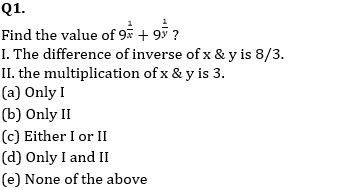Q2. How many women are required to complete the work in 12 days.
I. Efficiency of men are ⅔ rd of efficiency of women.
II. 16 men can complete the same work in 10 days.
(a) Only I
(b) Only II
(c) Either I or II
(d) Only I and II
(e) None of the above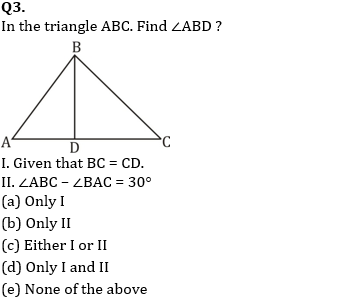Q4. What is the C.I. on a sum at the end of 3 years ?
I. C.I. at the end of two years is Rs. 110.
II. Difference between CI and SI at the end of two year is Rs. 100 and rate percent is 10%.
(a) Only I
(b) Only II
(c) Either I or II
(d) Only I and II
(e) None of the above

Q5. Two trains X and Y starts from point A and B respectively towards each other. What is the distance between them when they start ?
I. When both trains cross each other, time taken by train X to reach B is twice the time taken by train Y to reach A.
II. Distance between them after 60 min. is 800 km.
(a) Only I
(b) Only II
(c) Either I or II
(d) Only I and II
(e) None of the above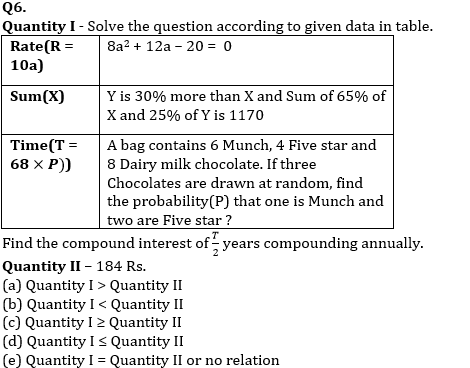Q7. Quantity I – Length of main diagonal of a cube is 60√3 cm, if cube is melted in the form of smaller cube with side of 4 cm each or cube is melted in the form of cuboids of each having length of 8 cm, width 5 cm & height 2 cm. Find the difference between total surface area of all smaller cubes & all cuboids formed (in m²)?
Quantity II – 3.48 m²
(a) Quantity I > Quantity II
(b) Quantity I < Quantity II
(c) Quantity I ≥ Quantity II
(d) Quantity I ≤ Quantity II
(e) Quantity I = Quantity II or no relation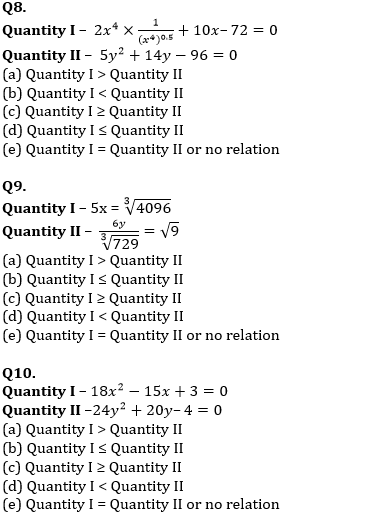Solutions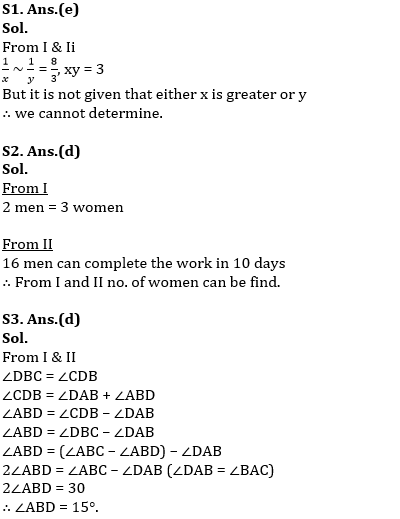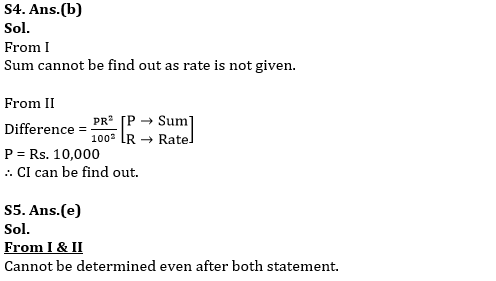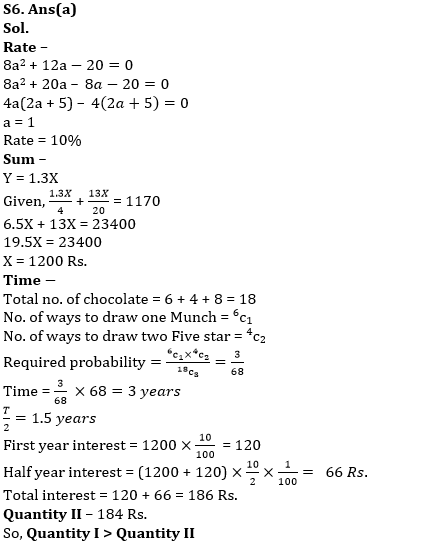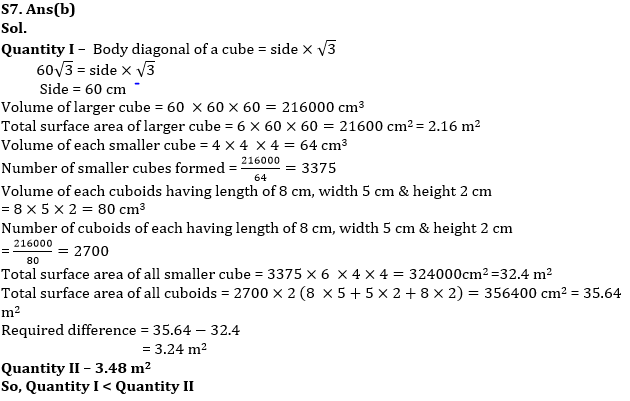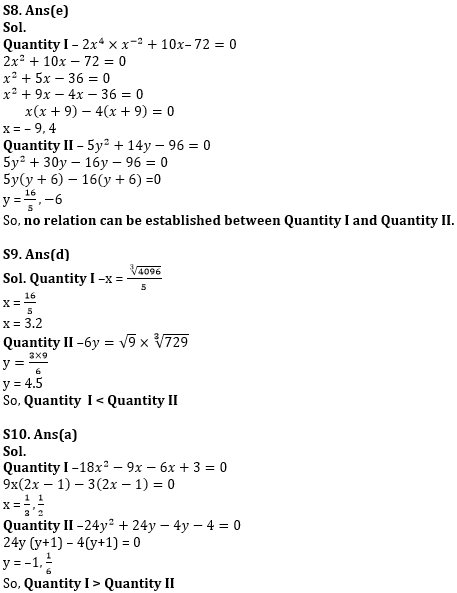#### Congratulations!Download Hindu Review of October 2021: Free PDF